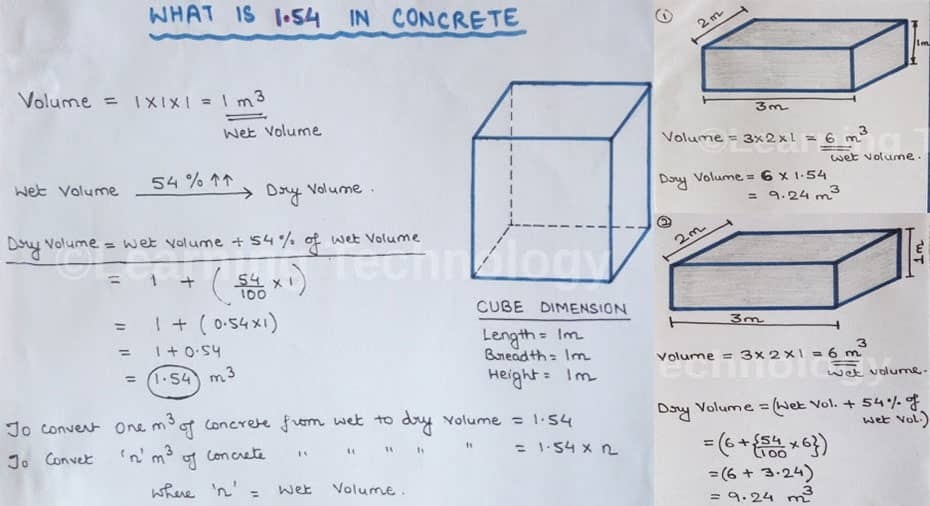Home Multimedia - Videos How to validate 1.54 in concrete

# How to validate 1.54 in concrete

In this civil engineering video tutorial, you will learn how 1.54 in concrete is derived for measuring the quantities of sand, cement and aggregate as well as making calculation of wet volume to dry volume.

Suppose, the dimension of the cube is taken as follow:-

Length = 1m, Breadth = 1m, Height = 1m

Volume = length x breadth x height = 1m3

It is the weight volume of the cube.

The quantity of concrete is determined on the basis of dry volume.

So, weight should be converted to dry volume. It is known that wet volume is increased to54% for being converted to dry volume.

Dry volume = wet volume+ 54% of wet volume

= 1 + (54/100 + 1) = 1 + (0.54 x 1) = 1+0.54 = 1.54 m3

To convert 1m3 of concrete from wet to dry volume = 1.54

To convert ?n? m3 of concrete from wet to dry volume = 1.54 x n

Here, ?n? denotes wet volume

Given below a rectangular cuboid and its dimension are as follow:-

Length = 3m, Width = 2m and Height = 1m

Volume = 3 x 2 x 1 = 6m3 = wet volume

When the water is added to cement, the volume of cement is decreased and for this reason the wet volume is converted to dry volume.

So, dry volume = 6 x 1.54 = 9.24m3

The above is validated with the following rule:

Volume = 3 x 2 x 1 = 6m3 = wet volume

Dry volume = (Wet volume + 54% of wet volume)

= (6 + 54/100 x 6) = (6 + 3.24) = 9.24 m3

##### To view the graphical demonstration of the above theory, go through the following video tutorial.

Video Source: L & T - Learning Technology

##### How to validate 1.54 in concrete